• 基于广义互相关的声源定位算法，matlab语言实现。
•matlab
• 使用matlab 实现广义互相关算法，得到时延求解
• 为了克服这问题，通过广义互相关算法（GCC）可提高这部分时差测量的准确性。广义互相关法将回波信号在频域进行加权处理，提高了信号频谱特性，抑制了噪声对测量的影响。实验结果表明，广义互相关算法在白噪声以及...
• 该代码为matlab代码，主要是利用广义互相关的方法计算两段信号的相位差
• 这是个matlab广义互相关计算时延的m文件，直接调用，十分方便，赶快下载啊，让我赚点积分，哈哈。matlab
• 基于EMD的广义互相关时延估计，孙书学，吕艳新，提出一种基于经验模式分解（Empirical Mode Decomposition，EMD）的广义互相关时延估计方法，用来解决信号非稳态且采集信号的各个通道间存�
• 宋老师，我把一个声音信号作了延迟之后加上噪声，用PHAT加权和基本互相关都能得到正确的时延值，但我对这两个信号进行小波分解和重构近似信号之后，用基本互相关可以得到时延，但PHAT加权后就不能得到正确的时延了，...

宋老师，我把一个声音信号作了延迟之后加上噪声，用PHAT加权和基本互相关都能得到正确的时延值，但我对这两个信号进行小波分解和重构近似信号之后，用基本互相关可以得到时延，但PHAT加权后就不能得到正确的时延了，这是为什么呢？下面附上代码和图。fs=2500/(t(end)-t(1));%采样频率 ，x是表格导入的数据，信号时间为t，长度是2500，这里上传不了表格
N=length(x);
x1=x-mean(x);%消除直流分量
x1=x1/max(abs(x1));%幅度归一化
%构造延时250个采样点，即延迟0.01S的信号x2
x2=[zeros(250,1);x1];
x2=x2([1:end-250],1);
x2=awgn(x2,15,'measured');
x1=awgn(x1,15,'measured');
%时延估计
X1=fft(x1,2*N-1);
X2=fft(x2,2*N-1);
X21=X2.*conj(X1);
R21=fftshift(real(ifft(X21)));%基本互相关
Ph21=fftshift(real(ifft(X21./abs(X21))));%PHAT加权
tt=(-N+1:N-1)/fs;%时间序列
figure(1)
subplot(2,1,1),plot(tt,R21),title('基本互相关');
subplot(2,1,2),plot(tt,Ph21),title('PHAT加权');
idx=find(R21==max(R21));  %峰值检测
t1=tt(idx)   %时延
%对x1,x2进行小波分解和重构
[c,l]=wavedec(x1,6,'db6');
[c2,l2]=wavedec(x2,6,'db6');
a16=wrcoef('a',c,l,'db6',6);    %wrcoef重构x1的逼近信号
a26=wrcoef('a',c2,l2,'db6',6);  %wrcoef重构x2的逼近信号
%对重构后的两个信号进行相关计算
A1=fft(a16,2*N-1);
A2=fft(a26,2*N-1);
A21=A2.*conj(A1);
% RA21=fftshift(real(ifft(A21)));%基本互相关
RA21=fftshift(real(ifft(A21./abs(A21))));%PHAT加权
figure(2)
plot(tt,RA21);
idx=find(RA21==max(RA21));  %峰值检测
t2=tt(idx)
figure(3)
subplot(2,2,1),plot(t,x1);title('原始信号x1');
subplot(2,2,2),plot(t,x2);title('延迟0.01s后的x2');
subplot(2,2,3),plot(t,a16);title('x1的近似重构信号');
subplot(2,2,4),plot(t,a26);title('x2的近似重构信号');
2019-8-13 23:03 上传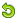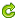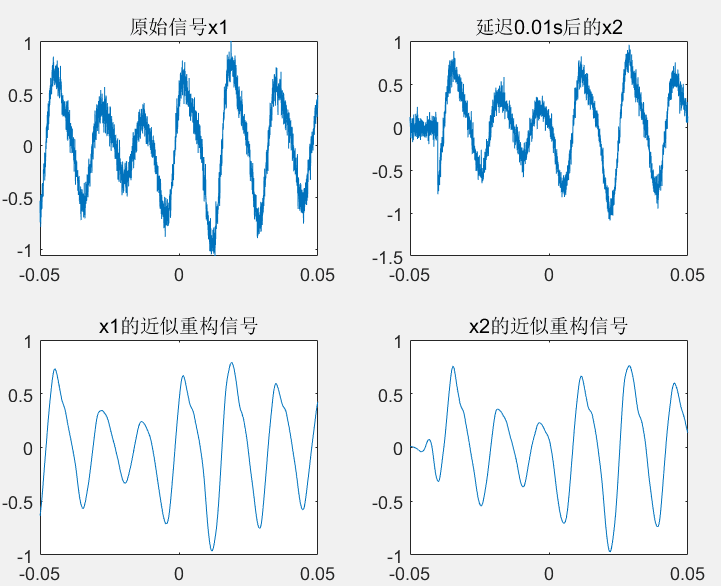2019-8-13 23:12 上传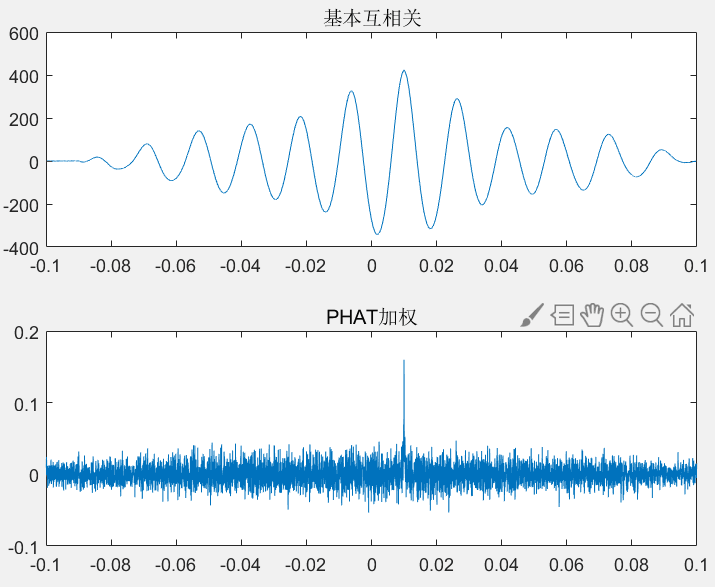2019-8-13 23:12 上传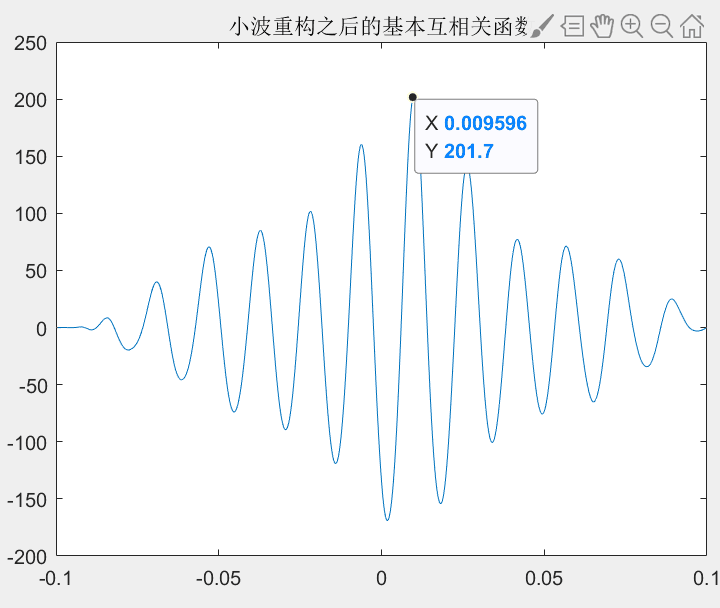2019-8-13 23:12 上传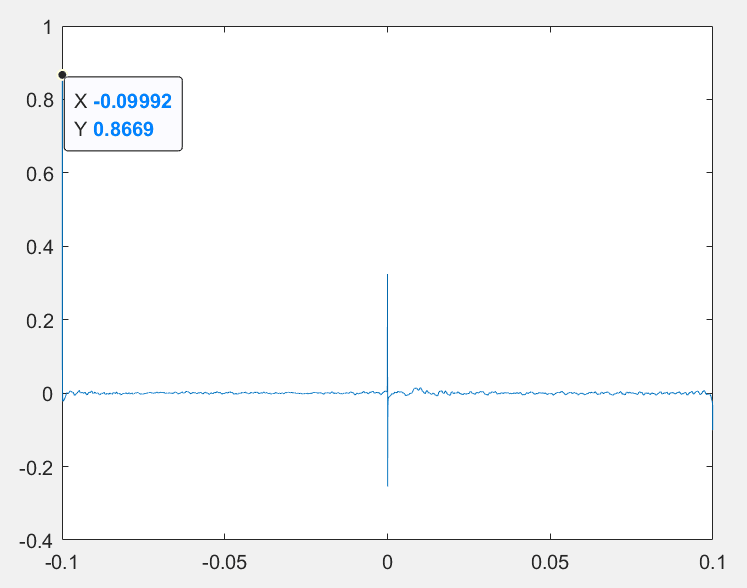展开全文• 读取音频，采用分帧处理，最后使用广义互相关算法求时延
• 基于广义互相关的声源定位算法，matlab语言实现。
• 基于TDOA定位的程序，利用广义互相关法获取时延估计GCC
•研究论文
• 基于FPGA的实时PHAT加权广义互相关时延估计器.pdf
• MATLAB加窗滤波在广义互相关时延估计中的应用.pdf
• 电子信息工程学院，长春 130022) 摘 要：针对麦克风阵列广义互相关声源定位方法中，麦克风接收到的人声信号掺杂混响以及噪声，导致算法求解过程中准确性不高的缺点，通过MATLAB分窗滤波的方法，在算法中对实时接收的...
长春理工大学学报(自然科学版) Journal of Changchun University of Science and Technology(Natural Science Edition) Vol.39 No.6 Dec.2016 第39卷第6期 2016年12月 收稿日期：2016-09-23 作者简介：王森(1991-)，男，硕士研究生，E-mail：wangsen321@126.com 通讯作者：宫玉琳(1983-)，男，博士，讲师，E-mail：garrygong1983@126.com MATLAB加窗滤波在广义互相关时延估计中的应用 王森，宫玉琳，王春阳，李明秋，李建建 (长春理工大学 电子信息工程学院，长春 130022) 摘 要：针对麦克风阵列广义互相关声源定位方法中，麦克风接收到的人声信号掺杂混响以及噪声，导致算法求解过程中准确性不高的缺点，通过MATLAB分窗滤波的方法，在算法中对实时接收的声音信号进行预处理，针对人声频率进行改进，提升低信噪比状况下估计时延的准确性，完成人声声源定位的目的，并通过仿真实验验证了该方法的有效性。关键词：声源定位；分窗滤波；广义互相关中图分类号： TN912.3 文献标识码：A 文章编号：1672-9870(2016)06-0048-05 ApplicationofMATLABWindowingand FilteringinGeneralizedCross-correlationTimeDelayEstimation WANG Sen，GONG Yulin，WANG Chunyang，LI Mingqiu，LI Jianjian (SchoolofElectronicsandInformationEngineering，ChangchunUniversityofScienceandTechnology，Changchun130022) Abstract：Aiming at the shortcomings of the microphone array signal de-noising and the reverberation of the human voice signal received by the microphone in the generalized cross-correlation sound source localization method，this paper uses MATLAB to divide the windowing and filtering method，And the accuracy of estimating the time delay under the condition of low SNR is improved and to complete the task of estimating the localization of the human voice source. Simulation experiments havs been carried out to verify the effectiveness of the proposed method. Key words：sound source localization；windowing and filtering；generalized cross correlation 麦克风阵列对语音定位的方法，常常应用在智能机器人、通信、火警救援等众多领域［1］
展开全文• 该文以研究微弱瞬态电磁辐射信号的探测手段为目的,提出广义互相关与混沌序列预测相集成的检测算法。基于双天线测试和互相关信息估计方法,将低信噪比条件下非周期微弱放电信号的检测问题转化为周期性时延参数的估计...
• 通过对被动目标深度测量...为了满足作为被动目标深度测量关键环节的时延估计的高精度要求,研究了快速广义互相关时延估计算法,并通过了仿真试验。试验结果证明了该算法具有良好的实时性,能够得到较高的深度测量精度。
• 针对这一问题，结合相关峰精确插值算法（FICP），提出了一种基于二次相关改进的广义互相关时延估计算法。该算法通过二次相关，有效地降低噪声的干扰，再利用FICP，提高相关函数的分辨率。仿真实验表明，无论在低...
最近查了很多文献，也尝试了很多方法，可是还是没有找到一个绝好的方法求同一声源下相隔一定距离的两麦克风测得声音序列的延时值。 目前公认最好的求延时值的方法是广义互相关法代码如下
ay=xlsread('C:\Users\solid\Desktop\最新测试\o1.xlsx','B2:B4002');
afs=8000;%采样频率
N=max(size(ay));%采样数
[R1,a1]=xcorr(ax,ay);%原始数据用互相关法求延时
[Rmax1,Rloc1]=max(R1);
t1=abs((Rloc1-N)/afs)

局限在互相关法有时会出现延时值不准的情况。而且大多数情况下声源为混频信号时延时值相较于更加准确。 因此尝试采用对两麦克风采集到的信号使用快速傅里叶变换，变换后最大幅值所对应频率可以更好的还原原信号，对此频率下两信号的相角做差，再换算为一周期内的延时信号。采用此方法算得延时值相对更加准确一些（尤其是对单频1KHz情况）因为1khz单频信号在频域下两信号最大幅值所对应的频率数值相同，但是混频时理论上是相同，可实际上总是不一样，因此混频下无法找到同一个最大幅值对应的频率。故此方法搁浅。代码就不放了有有兴趣的同学咱们私聊（q：530956317）然后想着试试对混频信号进行滤波试试，目前只用了一种滤波器butterworth滤波器。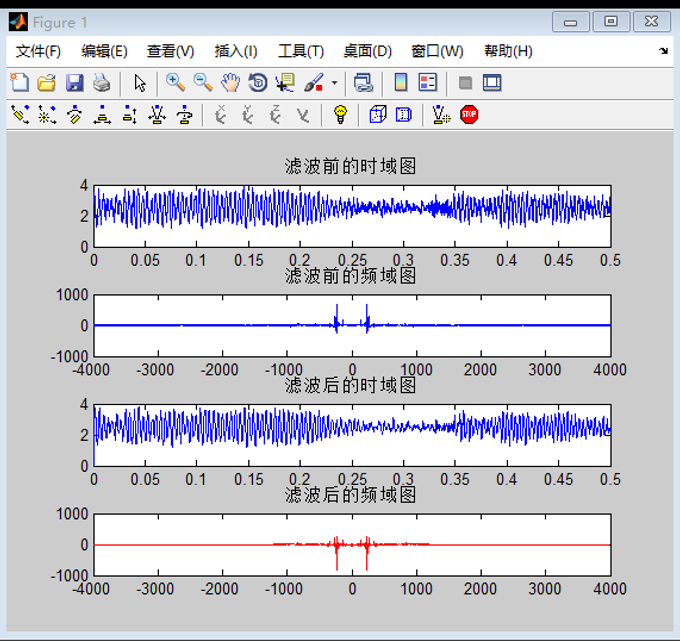最后，我又尝试了通过平移序列的方法找到相关系数最大的点，想着能够通过相关系数对延时值进行修正，结果时单频信号延时值越准却，平移序列后最大相关点出的相关系数值就越大。可是混频信号的数值飘忽不定又让我想到了放弃这种方法

N=4000;%采样数
fs=8000;%采样频率
A=[];
A(1)=abs(corr(x1, x2, 'type' , 'kendall'));
A(2)=abs(corr(x1(2:end), x2(1:end-1), 'type' , 'kendall'));
if A(1)<A(2)
for i=3:1:10
A=[A,abs(corr(x1(i:end), x2(1:end-i+1), 'type' , 'kendall'))];
if A(i)>=A(i-1)
i=i+1;
else
break
end
end
else
for i=2:1:10
B(1)=abs(corr(x1, x2, 'type' , 'kendall'));
B=[B,abs(corr(x2(i:end), x1(1:end-i+1), 'type' , 'kendall'))];
if  B(i)>=B(i-1)
i=i+1;
else
break
end
end
end
t2=(i-2)*(1/fs);
C=[];
for j=15: -1 :2
C=[C,corr(x2(j:end), x1(1:end-j+1), 'type' , 'kendall')];
end
for j=1: 1 :15
C=[C,corr(x1(j:end), x2(1:end-j+1), 'type' , 'kendall')];
end
D=-14: 1 :14;
stem(D,C)
title('平移后两信号相关系数');
[序列0+为x1向后移，x2向前移。序列0-为x2向后移，x1向前移

[序列0+为x1向后移，x2向前移。序列0-为x2向后移，x1向前移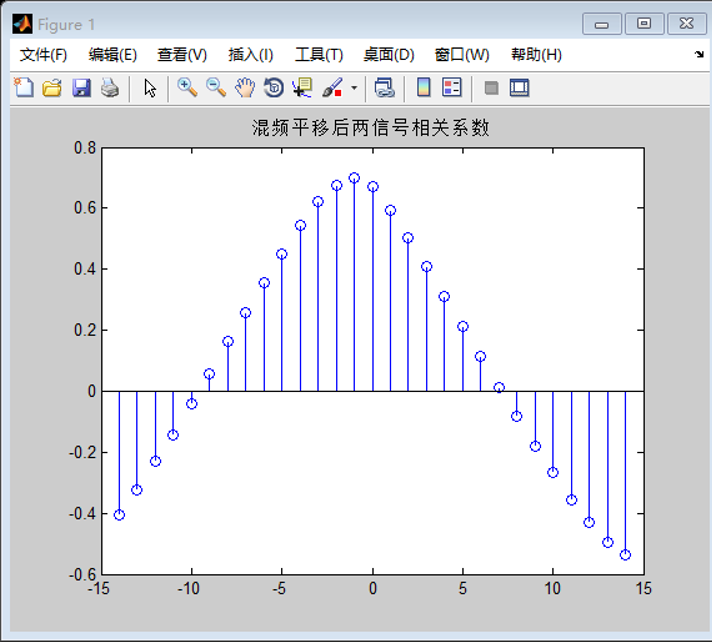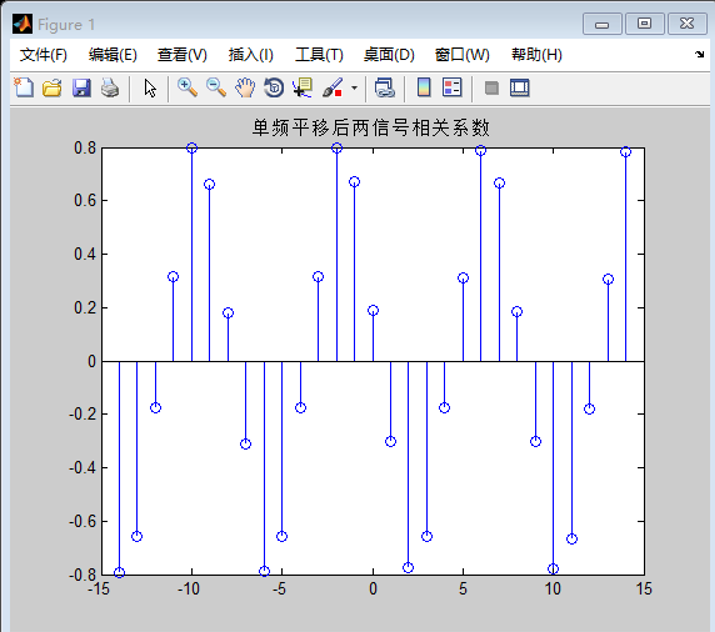可以看出相关系数还是呈现一定的周期变化的。 还是要继续努力寻找更好的求延时的方法。 哎，不得不吐槽一下声音信号的微弱性，很容易就测得不准确了。 在下研一小白一枚，有什么不准确的地方欢迎批评指正。欢迎交流分享。 谢谢大家
展开全文• 广义互相关算法如何用C语言实现? 在声音测距的原理中，我们可以利用麦克风对采集的声音信号利用互相关算法测出音源与麦克风距离的差值，而大致求出音源的方位。这也是第十五届智能车竞赛声音信标组寻灯的基础，因为...
广义互相关算法用FFT加速的C语言实现
在声音测距的原理中，我们可以利用麦克风对采集的声音信号利用互相关算法测出音源与麦克风距离的差值，而大致求出音源的方位。这也是第十五届智能车竞赛声音信标组定位的基础，因为我参加的是这个组别，所以当时在这方面找了很多相关的资料互相关的原理基本上懂，但是用C语言实现起来感觉和书本上的知识结合不了，但是真的运气好，试了很多很多次，终于写出来了，可能是乐于分享的人运气都不会太差吧，O(∩_∩)O哈哈~
小说明：我的这个代码不是完整的工程，只是把核心的互相关算法及一些相关的代码分享出来了，是基于单片机的，具体的实践还要靠大家自己来结合，这个对于大家应该就是小意思了吧，哈哈。在其中我是用了FFT加速，因为采集的数据比较多，不用FFT加速的话会花费大量的CPU的处理时间，至于FFT算法，网上有很多，我这里就不写了。
//采样点数和FFT运算的点数最好都为2的N次方，且FFT_Pointer =2*Sampling_Num
#define Sampling_Num 2048
#define FFT_Pointer 4096

int Mic12_Delay;
int Mic34_Delay;
uint Max_Array_Num=0;            //互相关运算得到的最大值对应的数组下标
//以下这些参与FFT运算的数组占用的内存相当大(6*4*2*4096=192K),为了减小内存的话可以相互覆盖掉，我这里是为了让大家明白各个数组的作用
cfloat32 Mic1_fftIn[FFT_Pointer];//麦克风Mic1参与FFT的时域输入数组
cfloat32 Mic2_fftIn[FFT_Pointer];//麦克风Mic2参与FFT的时域输入数组
cfloat32 Mic1_fftOut[FFT_Pointer];//Mic1经过FFT之后得到的频域输出数组
cfloat32 Mic2_fftOut[FFT_Pointer];//Mic2经过FFT之后得到的频域输出数组
cfloat32 Mic1_ifftMul[FFT_Pointer];//FFT运算之后两个信号频域的乘积
cfloat32 Mic_ifftOut[FFT_Pointer];//最终经IFFT运算(FFT的逆变换)之后得到的时域信号
//定义浮点型复数的实部和虚部部分，因为FFT过程中既有实数也有复数
typedef struct
{
float real;               /**< \brief Real part */
float imag;               /**< \brief Imaginary part */
} cfloat32;
//两个复数相乘
cfloat32 mul(cfloat32 a, cfloat32 b)
{
cfloat32 c;
c.real = a.real*b.real - a.imag*b.imag;
c.imag = a.real*b.imag + a.imag*b.real;
return c;
}

//复数求出幅值
float Absolute_Value(cfloat32 a)
{
float i,j;
float value;
i=a.real*a.real;
j=a.imag*a.imag;
value=i+j;
return value;
}
/*********************************************************
********************* 核心函数 **************************
* 函数功能：1.Mic1和Mic2采集麦克风数据
*          2.利用FFT通过互相关算法求出时延差
********************************************************/
{
uint i;
uint array;
float MIC_ifftOut[FFT_Pointer];   //互相关运算的结果的模，因为结果可能为复数，参与运算要化为实数
float Mic_max=0.0;
for(i=0;i<Sampling_Num;i++)
{
Mic1_fftIn[i].imag               =0;    //虚部全部为0
Mic1_fftIn[i+Sampling_Num].imag  =0;    //虚部全部为0
Mic2_fftIn[Sampling_Num-i-1].imag=0;    //虚部全部为0
Mic2_fftIn[i+Sampling_Num].imag  =0;    //虚部全部为0

//将采集到的声音信号通过波形图实时显示
/*		array=(uint)Mic1_fftIn[i].real;
array=(uint)Mic2_fftIn[Sampling_Num-i-1].real+4096;
array=4096;
virtual_Osc_send_array(array,3);*/
}
FFT(Mic1_fftOut, Mic1_fftIn, FFT_Pointer);     //Mic1的FFT正变换
FFT(Mic2_fftOut, Mic2_fftIn, FFT_Pointer);     //Mic2的FFT正变换
for(i=0;i<FFT_Pointer;i++)
{
Mic1_ifftMul[i]=mul(Mic1_fftOut[i],Mic2_fftOut[i]);      //两个信号频域的乘积
}
IFFT(Mic_ifftOut, Mic1_ifftMul, FFT_Pointer);  //FFT的逆变换
for(i=0;i<FFT_Pointer;i++)
{
MIC_ifftOut[i]=Absolute_Value(Mic_ifftOut[i]);           //取模值,因为经过IFFT之后得到的可能为复数，不好比较大小，所以通过取模值化为实数
}
for(i=0;i<FFT_Pointer;i++)
{
if(MIC_ifftOut[i]>Mic_max)
{
Mic_max=MIC_ifftOut[i];            //找出幅值最大的点,此即为相关性最好的点
Max_Array_Num=i;                   //相关性最好的点对应的数组下标
}
}
//Max_Array_Num为基准线,通过比较Sampling_Num-1在Max_Array_Num的上下方得出音源距离Mic1与Mic2距离的差值
Mic12_Delay=Max_Array_Num-Sampling_Num+1;  //得到时延的下标差

//山外虚拟示波器画图,发送函数在我的另一篇博客串口发送中有介绍
/*	array=Max_Array_Num;
array=Sampling_Num-1;
virtual_Osc_send_array(array,2);*/
}

好了，以上就是利用互相关算法求时延差的C语言算法，代码的备注我已经尽可能的加在相应代码之后了，如果大家还有不懂的欢迎下方留言或者私信给我，尽量我懂的地方让大家也能懂！或者有什么不对的地方欢迎大家指出，我们共同进步！展开全文算法 c语言 单片机
• ## 广义互相关计算TDOA

千次阅读 多人点赞 2020-06-05 23:46:14
你可以使用广义互相关（ Generialized Cross-Correlation ）来估计一个信号达到两个传感器的时间延迟。比如应用麦克风阵列来确定声源方位的应用背景。问题可以用以下模型来描述： r1(t)=s(t)+n1(t)r_1 \left( t \...算法
• 在网络上有一篇关于 基于广义互相关估计时间延时 的PDF文件，是由Bhargava提交一篇综述报告，将用于估计时间延迟的广义互相关算法进行了总结。   ■ 互相关估计时延 在空间上相距一定距离LLL的两个声音传感器...
• 看了国内外的文献，一致首推“广义相关（Generalized Cross Correlation–GCC）算法”。 查文献，在matlab中使用模拟正弦波仿真测试了一下广义相关算法，没有达到预期效果，百思不得其解。浏览了一下网络上关于GCC的...matlab 自动驾驶 算法...